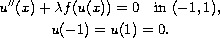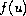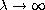Sixth Mississippi State Conference on Differential Equations and Computational Simulations.
Electron. J. Diff. Eqns., Conference 15 (2007), pp. 239-249.

### On the exact multiplicity of solutions for boundary-value problems via computing the direction of bifurcations Joaquin Rivera, Yi Li

Abstract:
We consider positive solutions of the Dirichlet problemdepending on a positive parameter. We use two formulas derived in  to compute all solutionswhere a turn may occur and to compute the direction of the turn. As an application, we consider quintic a polynomialwith positive and distinct roots. For such quintic polynomials we conjecture the exact mutiplicity structure of positive solutions and present computer assisted proofs of such exact bifurcation diagrams for various distributions of the real roots. The limiting behavior of the solutions on these bifurcation branches asand their stabilities are also investigated.

Published February 28, 2007.
Math Subject Classifications: 34B15.
Key Words: Bifurcation points; direction of the turn; multiplicity of solutions.

Show me the PDF file (252K), TEX file, and other files for this article.Joaquin Rivera Department of Mathematics, University of Iowa Iowa City, Iowa 52242, USA email: rvera@math.uiowa.edu Yi Li Department of Mathematics, University of Iowa Iowa City, Iowa 52242, USA. Hunan Normal University Changsha 410081, Hunan, China email: yi-li@uiowa.edu